# CH 610A/618A

First Exam

Fall, 2002

Prof.N.L.Bauld

__________________________________________                                                                              Name (Last, First)

__________________________________________                                                                            Social Security Number

## Possible Points

Page/Pts/Points Earned

## Score

I.

22

2/13/

3/9/

II.

23

4/10/

5/10/

6/3/

III.

20

7/10/

8/10

IV.

16

9/9/

10/7/

V.

19

11/11/

12/8/

Totals

100

### I.Ground State Electronic Configurations and Hybridizations

A.  Electronic Configurations.

1.   [2 pts] Write the ground state electronic configuration of atomic nitrogen (7N) in both the linear and energy level diagram formats.

Linear Format: 1s22s22px12py12pz1

Energy Level Diagram Format: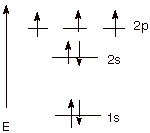2.   [4 pts] Name the three rules which are needed to derive the correct ground state configuration and explain how they are applied to the case of nitrogen.

1. Aufbau Principle—fill electrons in beginning with lowest energy AO and progressing to higher energy orbitals, i.e., first 1s, then 2s, then 2p.

2. Pauli Exclusion—maximum of 2 electrons per orbital, these having spins paired.

3. Hund Rule—where degenerate orbitals (orbitals of equal energy) are involved, populate each degenerate orbital with one electron before any orbital is doubly occupied. The lowest energy state is the one with all of the electron spins parallel, i.e., unpaired. Thus, each of the three 2p AO’s has one electron, and they all have their spins oriented in the same sense.

3.   [1 pt] Explain in a more fundamental way than just citing a certain rule why the three highest energy electrons are distributed to orbitals as they are.

Placing electrons in different orbitals tends to minimize their repulsions, since they are on average further apart than when they occupy the same orbital.

4.    [1 pt] Explain in a more fundamental way than just citing a certain rule why these same three electrons are assigned the spins as they are correctly assigned.

5.

Electrons which have the same spin are less repulsive toward each other than paired electrons.

B.  Electronic Configuration and Hybridization of Carbon.

1.   [1 pt] Using the linear format only, write the ground state electronic configuration of carbon atoms (6C).

1s22s22px12py12pz0

2.   [4 pts] Starting with the ground state electronic configuration of carbon, show in stepwise fashion (two steps) how carbon atoms can hybridize to the sp3 hybridization state. Be sure to include (1) the names of any other states involved in addition to the ground state (2)the potential valence of carbon in that state and (3)comment on the energetic feasibility of each of the individual steps and of the overall process of hybridization to the sp3 state.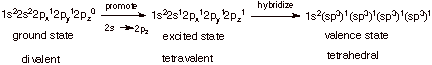q      The first step is about 100 kcal/mol endergonic, since the 2p AO is of substantially higher energy than the 2s AO.

q      The second step costs nothing energetically, but expands the valence from 2 to 4, and is therefore about 200 kcal/mol exergonic (ca. 100 kcal per bond).

q      Overall, the process is about 100 kcal/mol exergonic, i.e., favorable.

C.  Atomic Ions.

1.    [1 pt] There is one positively charged ion and one negatively charged ion which has the same ground state electronic configuration as atomic carbon. What are these ions?

N+  and B- (or 7N+ and 5B- ).

2.    [2 pts] What is the name of the principle which interrelates atomic carbon and these two atomic ions? What does this principle predict about the hybridization state of these atomic ions when bonded to four hydrogen atoms?

q      The Isoelectronic Principle

q      They have the same hybridization state (all sp3).(Or, the same valence angles or the same geometrical shape)

D.  Carbocations.

1.    [2 pts] Write the ground state electronic configuration of positively charged carbon atoms (6C+), and show, in stepwise fashion  how the typical hybridization state of carbocations (trivalent, positively charged carbon) is derived. What hybridization state is this?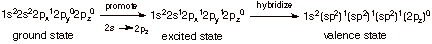q      This is the sp2 state.

2.    [1 pt] What neutral atom has the same hybridization state as positively charged carbon?

q      Boron

E.  Alkenes

1.    [2 pts] Starting with the ground state electronic configuration of neutral carbon, sketch the stepwise development of the hybridization state of carbon in ethene and other alkenes. What is the designation of this state?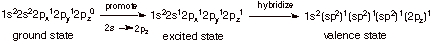q      This is also the sp2 hybridization state.

2.   [1 pt] When (under what general circumstances) does neutral carbon adopt this hybridization state?

q      When it is bonded to 3 other atoms.

### II. Atomic Orbitals, Molecular Orbitals, and Bonding

#### A. Carbon-Carbon Bond Formation

1. The overlap of two carbon sp3 AO’s can result in the formation of a strong, covalent carbon-carbon single bond.

a.   [3 pts] Referring to the diagram given below, label the two new orbitals which are formed when these two sp3 orbitals overlap, providing both the abbreviations and the full names of the orbitals. Explain the basis for the names assigned to them.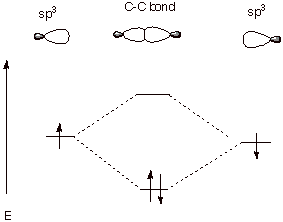q      The MO of lower energy is designated as a BMO, i.e., a bonding molecular orbital).

q      The higher energy MO is designated as an ABMO or an anti-bonding molecular orbital.

q      The lower energy MO is more bonding (i.e., lower in energy) than the original sp3 atomic orbital. The higher energy MO is less bonding (higher in energy) than the original atomic orbital.

b.   [2 pts] Explain why the two electrons both go into the same orbital. According to what principle must their spins be paired?

q      For one electron to go into the ABMO would make its energy much higher. This is essentially the Aufbau principle at work.

q      The Paul Exclusion Principle requires that electrons in the same orbital be different, i.e., have different spins.

c.   [2 pts] Given the geometric nature of the overlap depicted in the diagram above, what kind of covalent bond is this? What kind of overlap is involved in this kind of bond?

q      A sigma bond.

q      End-on overlap (or some equivalent term).

d.   [3 pts] Provide a depiction of both  of the new orbitals which are formed in the bond formation process indicated above in terms of their constituent sp3 orbitals. In terms of a specific type of overlap, what is different about the low energy orbital and the high energy orbital?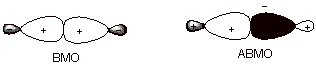q      The BMO has positive overlap, i.e., overlap of orbital lobes of the same sign, whereas the ABMO has negative overlap, i.e., overlap of orbitals of opposite sign.

2. A bond line structure for ethene is given below. The following questions refer to the bonding in ethene.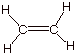a. [2 pts] Provide an orbital depiction of each of the sigma bonds in ethene (carbon-hydrogen and carbon-carbon).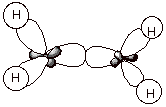b. [2 pts] Provide an orbital depiction of the second carbon-carbon bond present in ethane. What kind of bond is this? What kind of overlap (geometrically) is involved?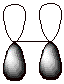q      This is a pi bond.

q      Pi overlap occurs laterally (or in a sidelong fashion)

B. Strong and Weak Covalent Bonding.

1.   [3 pts] Specify the general requirements for strong covalent bonding, briefly explaining the basis for each requirement.

q      Efficient net overlap

q      Two paired electrons

2.   [3 pts] Cite two specific situations in which atoms closely approach and efficient orbital overlap results, but no covalent bonding at all results. The situations should be fundamentally different. Provide energy level diagrams illustrating each of these cases.

Case I. Dihelium, formed from two helium atoms. Each helium atom has 2 electrons in the 1s AO, so four electrons must be filled in. Two in the bonding MO and two in the ABMO, the antibonding exactly nullifies the bonding.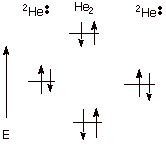Case 2. Two hydrogen atoms each of which have one electron in a 1s AO, but the electrons have the same (unpaired) spin. Since two electrons cannot go into the same BMO, one must go into the BMO. Again, antibonding nullifies bonding.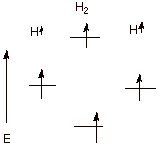3.   [3 pts] Cite two specific situations in which atoms closely approach and efficient orbital overlap occurs, but only weak or modest covalent bonding results. Again, the two situations should be fundamentally different. Provide energy level diagrams illustrating each of these cases.

q      Case I. One electron bonding. Example: A proton combines with a hydrogen atom. Only one electron is present and is able to go into the BMO providing some bonding, but not as much as when there are two electrons in the BMO.

q      Case 2. Three electron bonding. Example: A hydride anion combines with a hydrogen atom. There are three electrons and two can go into the BMO, while one must go into the ABMO. There is net, but weak bonding.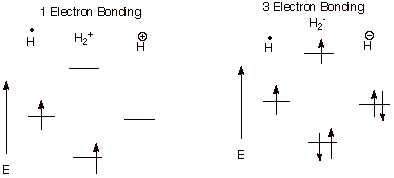### III. Nomenclature, Formal Charges, and Resonance Structures

A.  Formal Charges.

1.   [5 pts] Calculate the formal charges on each atom other than hydrogen in the following structures. Provide the formula you are using, and show your calculations. Then, calculate the net charge on the species.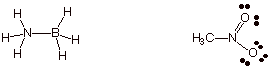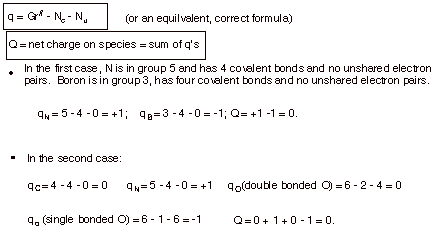B.  Resonance Structures.

1.   [1 pt] Explain why the following structure is not an acceptable canonical or resonance structure. Do not merely cite the octet rule, but explain in a more basic manner, involving orbitals and electrons which would have to be involved in the second N-B bond.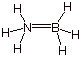q      To form a second N-B bond, one of these atoms would have to have a filled AO in the valence shell (presumable B, since it is negatively charged in the best valence structure) and one would have to supply a vacant orbital (presumably N, since it is positively charged in the best canonical structure). Neither has either a vacant or a filled  orbital available to bond, since both have already used all four valence shell AO’s to form the four covalent bonds.

2.   [2 pts] Using the appropriate formalism of resonance theory, describe the acetate ion (one canonical structure is shown below) in terms of resonance theory.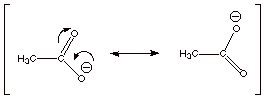3.   [1 pt] Summarize this as a dotted line/partial charge structure.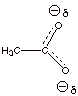4.   [1 pt] The resonance energy of the acetate anion is characterized as large. Upon what basic principle of resonance theory is this based?

q      Resonance stabilization is large when canonical structures are of equal (or even nearly equal) energy.

5.   [1 pt] What is the main geometric consequence of resonance in the acetate anion?

q      Both C-O bonds are of the same length and are intermediate between single and double.

6.   [1 pt] Provide an depiction of the pi electron system of the acetate anion. Such a pi electron system is termed a conjugated system. Over how many atomic orbitals are the MO’s of this conjugated system delocalized?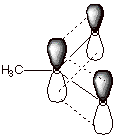q      Over three atoms, carbon and two oxygens.

C. Nomenclature

1. [3 pts each = 6 pts] Provide complete, accurate IUPAC names for the following molecules. As a part of your answer, include the numbering system and the basis for your choice of numbering system.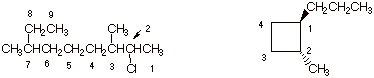q      The molecule on the left is designated: 2-chloro-3,7-dimethylnonane. Numbering from the right side gives the first substituent (Cl) at position 2, whereas numberinig from the left would have given the first substituent (methyl) at position 3. Of course the longest continuous chain must include the ethyl group at C8 and C9.  Chlorine is placed before dimethyl because of alphabetics.

q      The cycloalkane is: trans-1-methyl-2-propylcyclobutane. Since the ring has four carbons and the largest substituent only three, it is named as a cyclobutane derivative. Methyl is listed first because of alphabetics, and this substituent defines the 1 position of the ring for the same reason.

2. [2 pts] Provide the IUPAC name for the following substituent: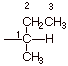q      Numbering begins at the unspecified valency and continuous along the longest continuous chain. The name is therefore 1-methylpropyl.

IV. Conformational Analysis; Torsional Strain; Steric Effects.

A.  Conformational Analysis of Ethane.

1.   [3 pts] Sketch an energy vs. dihedral angle conformational energy plot for ethane, providing the names, Newman projection structures, and relative energies of the two conformational extrema (minimum and maximum).

q      Note that I have not drawn a curve connecting the eclipsed and staggered forms because my software won’t do that. It is expected that you would draw a smooth curve connecting the points, as we have done in class.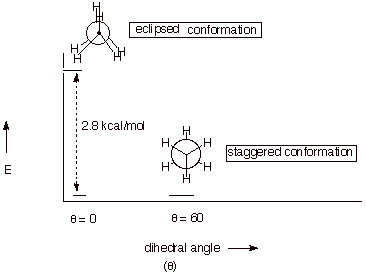B.  Conformational Analysis of Butane

1.   [4 pts] Sketch an energy vs. dihedral angle conformational energy plot for butane, again providing the names, Newman projection structures, and relative energies of all conformational extrema.2.   [1 pt] What is the name of the type of effect which accounts for the difference in energies of the two energy minima of butane. Describe the fundamental basis for this effect.

q      Steric effect or van der Waals repulsion.

q      A repulsion between hydrogen atoms of the methyl groups which are too close, i.e., closer than the sum of the van der Waals radii.

2.   [2 pts] Explain the difference between torsional strain and steric strain , using a depiction to assist in illustrating your answer.

q      Steric strain derives from a repulsion between atoms which are too close, while torsional strain derives from the repulsions of electron pairs in bonds, which are closer in the eclipsed than in the staggered conformation.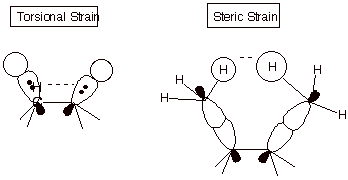C.  Ring Strain.

1.   [3 pts] Using the diagram provided below as a starting point for your illustration, describe and illustrate the basis for the angle strain present in cyclopropane. Be certain to include the concepts of interorbital angle and internuclear angle in your explanation.

q      The internuclear angle for an equilaterial triangle is 60­­ degrees, but the interorbital angle for sp3 orbitals on the same atom is the tetrahedral angle.

q      The result of this non-correspondence is that the orbitals on the ring carbons can not point toward each other, as is the case for a typical, strong carbon-carbon sigma bond. The bond is “bent”.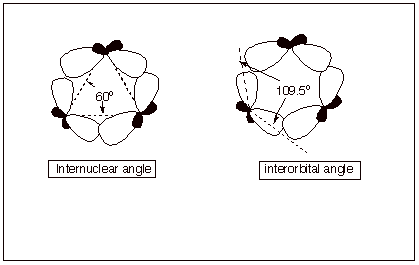2.   [2 pts] What other type of strain is present in cyclopropane? Explain the basis for this strain, and indicate which bonds are responsible for it.

q      Cyclopropane also has torsional strain because, being planar, all of the C-H bonds are eclipsed.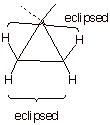V. Conformations of Cyclohexane and Substituted Cyclohexanes.

A. Cyclohexane Conformational analysis.

1. [2 pts] Draw the conformational structure of the conformation of cyclohexane which represents the energy minimum. Show clearly and label the axial and equatorial carbon-hydrogen bonds.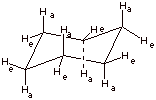2. [3 pts] How much strain is present in this conformation of cyclohexane? Explain your answer in detail, with reference to both types of strain which are typically present in cycloalkanes.

q      Zero strain.

q      There is no torsional strain because all of the C-H and C-C bonds are staggered.

q      There isi no angle strain because all of the C-C-C angles are 109.5 degrees.

3. [1 pt] Draw the structure of this energy minimum conformation again and then show (connected by a equilibrium arrows) the structure which would result upon “ring flip”. (Do not show the detailed conformational path at this point, but just the final ring flip structure.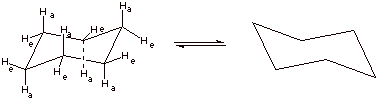4. [2 pts] Draw and name the structure of the global energy maximum on the ring flip path, and indicate its energy relative to the minimum energy structure. What is the importance of this structure?q      This is the “half chair” conformation.

q      Its energy is 10 kcal/mol greater than that of the chair.

B. Monosubstituted Cyclohexanes.

1.    [3 pts] Draw the conformational structure of the conformation of methylcyclohexane which is of lowest energy and show the structure which results upon ring flip. Provide the names and relative energies of these two structures.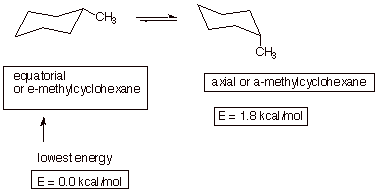2. [2 pts] Using a depiction to assist in clarifying your answer, explain which structure is the global minimum, what type of strain is present in the less stable structure, and the basis for the strain.

q      As noted, the equatorial conformation is more stable.

q      The axial conformation has steric strain or diaxial strain. Alternatively, it can be described as the equivalent of two gauche butane interactions.

q      This arises from the hydrogens of the methyl group repulsing each of the other two axial hydrogens on the same same of the generalized plane.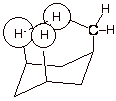2.    [3 pts] Arrange the following monosubstituted cyclohexanes in order of their relative amounts of equatorial conformer (1 = the substance which has the highest amount of equatorial isomer) and explain your chosen order.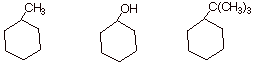q      Tert-butylcyclohexane, the third molecule, has the least axial and therefore the most equatorial (it is #1). This is because of the large steric size of the tert-butyl group, which makes the axial conformation highly strained.

q      Methylcyclohexane, the first molecule, is #2.

q      Cyclohexanol, the second molecule, is number 3, i.e.,it has the least amount of equatorial conformation, because the OH group is relatively small and the axial conformation, though still less stable than the equatorial, is less strained than either the methyl- or tert-butylcyclohexane.

C. Disubstituted Cyclohexanes.

1.    [1 pt each = 3  pts] Specify quantitatively how much strain is present in each of the following disubstituted cyclohexanes and indicate what type of strain it is.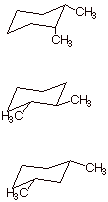q      The first molecule, cis-1,2-dimethylcyclohexane has 2.7 kcal/mol of strain. This derives from, first, an axial methyl, which engenders 1.8 kcal of strain and, secondly, from the two methyl groups which are gauche to one another. This adds another 0.9 kcal/mol of steric strain, analogously to the case of gauche butane.

q      The second molecule has 0.9 kcal/mol of strain. Though it has no axial methyl groups, the methyls are gauche toward one another.

q      No strain. Both methyls are equatorial and, being 1,3, are not close enough to have a gauche interaction.